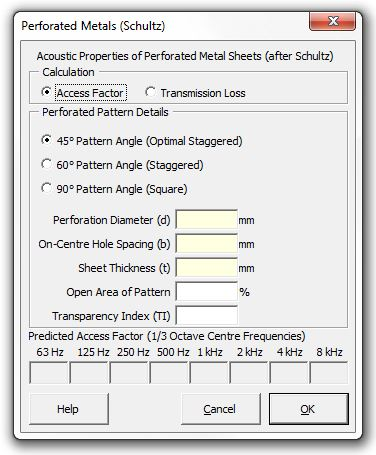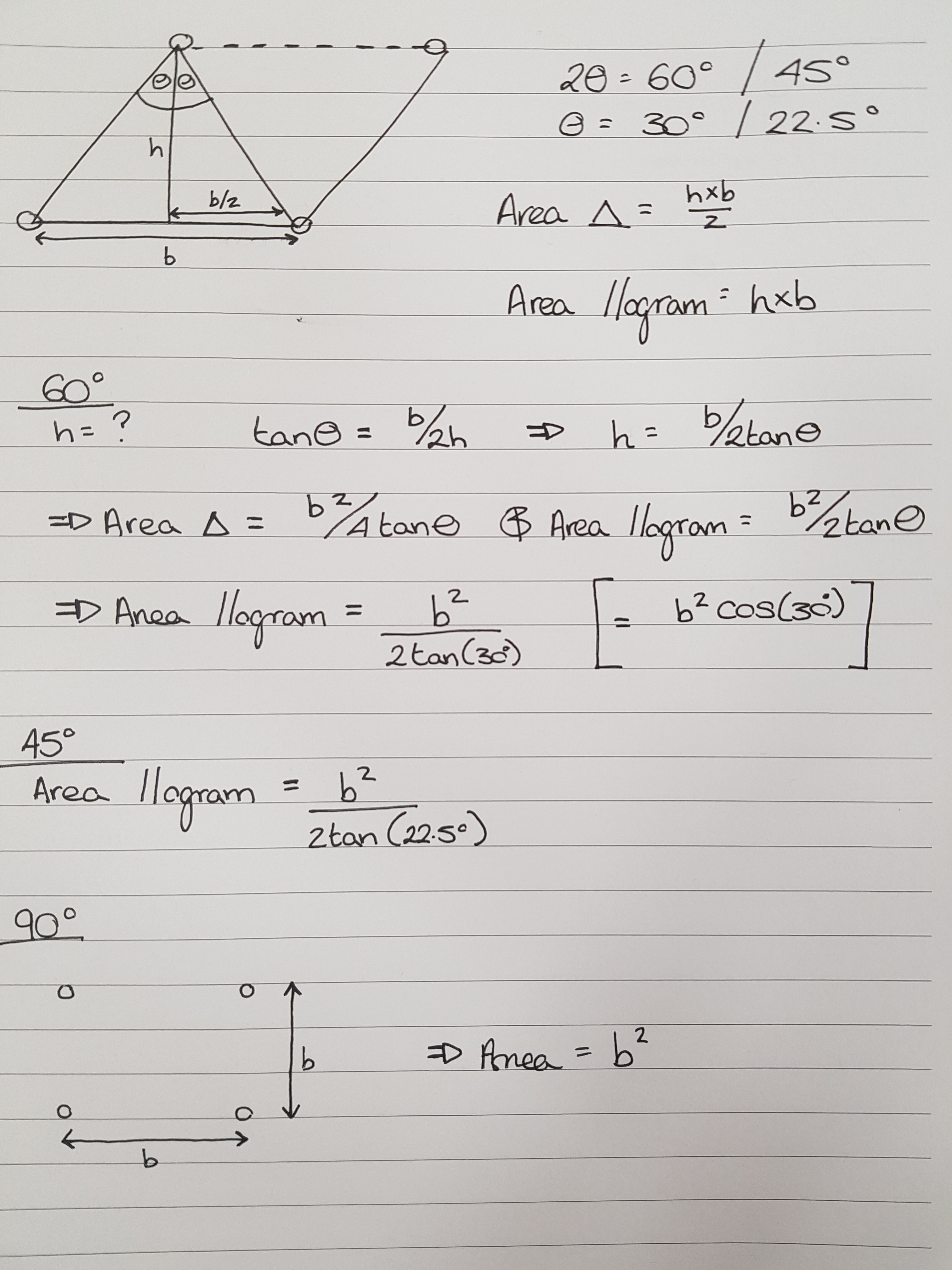﻿ Perforated Metals (Schultz)### Strutt Help

Acoustic Properties of Perforated Metal Sheets    1/1,1/3

Strutt|Building Acoustics|Perforated Metals (Schultz) calculates the acoustic properties of a perforated metal sheet and inserts either the access factor (AF) or the transmission loss (TL of the sheet into the current row of the workbook.The acoustic properties of a perforated metal sheet are quantified by the Transparency Index, which is calculated using the following formula:

TI = (nd^2)/(ta^2)

where:
n is the number of holes per square inch
d is the perforation diameter (in)
t is the sheet thickness (in)
a is the distance between holes
a = b-d;
b is the on-centre hole spacing (in)

The perforation pattern can be either 45° staggered,60° staggered or 90° square. Note that the 45° staggered pattern is the 90° pattern rotated; however the distance between holes is not the same - in the 90° pattern it is the shortest distance between holes, while in the 45° pattern it is the distance between holes in each row of the pattern:The third-octave band transmission loss and access factor values for the perforated metal sheet are determined by interpolating within the curves given in Appendix D of Schultz's guideline, based on the calculated Transparency Index for the perforated metal sheet.

Access Factor is the modification to the absorption coefficient of a material when the perforated metal sheet is installed in front of that material - e.g. if a material has an absorption coefficient of 0.8 at 4 kHz, and the perforated sheet has an access factor of 0.7, the absorption coefficient of the material covered with the perforated sheet would be (0.8*0.7)=0.56 at 4 kHz.

Reference: Theodore J Schultz, PhD (1986) Acoustical Uses for Perforated Metals: Principles and Applications